# Linear summation method

(diff) ← Older revision | Latest revision (diff) | Newer revision → (diff)

A summation method (cf. Summation methods) having the properties of linearity:

1) if the series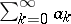is summable by the summation method to the sum, then the seriesis summable by this method to the sum;

2) if the series,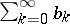are summable by the summation method toandrespectively, then the series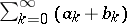is summable by this method to the sum.

All most widespread summation methods are linear; in particular, a matrix summation method and a semi-continuous summation method. There are non-linear summation methods. For example, the method in which summability of a series to the sumis defined by the existence of the limitof the sequence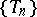, where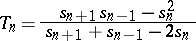(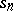are the partial sums of the series), is not linear.

How to Cite This Entry:
Linear summation method. Encyclopedia of Mathematics. URL: http://encyclopediaofmath.org/index.php?title=Linear_summation_method&oldid=17658
This article was adapted from an original article by I.I. Volkov (originator), which appeared in Encyclopedia of Mathematics - ISBN 1402006098. See original article